#Function Repository Resource:

# VariationalD

Get the variational derivative of a functional

Contributed by: Wolfram Research
 ResourceFunction["VariationalD"][f,u[x],x] returns the variational derivative of the integral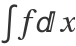with respect to u[x], where the integrand f is a function of u, its derivatives and x. ResourceFunction["VariationalD"][f,u[x,y,…],{x,y,…}] returns the variational derivative of the multiple integral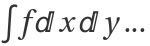with respect to u[x,y,…], where f is a function of u, its derivatives and the coordinates x,y,.... ResourceFunction["VariationalD"][f,{u[x,y,…],v[x,y,…],…},{x,y,…}] gives a list of variational derivatives with respect to u,v,....

## Examples

### Basic Examples

Variational derivative for the arc length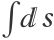of a plane curve:

 In:=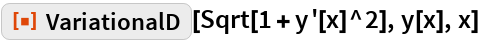Out=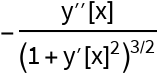Variational derivative for the Dirichlet integral in two variables:

 In:=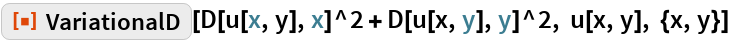Out=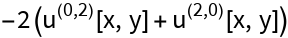## Requirements

Wolfram Language 11.3 (March 2018) or above